# Chapter 3.1. Simple Conditions

In the current chapter, we're going to be taking a look at the conditional constructs in the Python programming language. By implementing these constructs, our program can produce a different output based on a given specific input. We will explain the syntax of the conditional operators (if, if-elif and else) by implementing appropriate examples and also we are going to take a look at the range in which a variable lives (its scope). Finally, we will go over different debugging techniques, to follow the programming steps through which our program goes during its run.

## Number Comparison

In programming, we can compare values through the use of the following operators:

• Operator < (less than)
• Operator > (greater than)
• Operator <= (less than or equals)
• Operator >= (greater than or equals)
• Operator == (equals)
• Operator != (not equal; different than)

The result from a comparison is the so-called Boolean value – True or False, depending on the evaluated result being either true or false.

### Problems of Number ComparisonsNote that when printing true and false values in Python, they are capitalized, True and False, respectively.

### Comparison Operators

In Python, we can use the following operators to compare data:

Operator Notation Applicable for
Equals == numbers, strings, dates
Not equal !=
Greater than > numbers, dates, other comparable data types
Greater than or equal >=
Less than <
Less than or equal <=

Here is an example: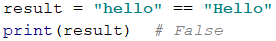## Simple If Comparisons

In programming, we often check particular conditions and perform various actions depending on the result of the comparison. This is done through if clauses, which have the following structure:

if condition:
# body of the conditional construct


### Problem: Excellent Result

We take the grade as an input in the console and check if it is excellent (≥ 5.50).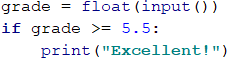Test the example code locally. Try entering different grades, for example, 4.75, 5.49, 5.50 and 6.00. For grades less than 5.50 the program will not give any output, however for grades of 5.50 or greater, the output will be "Excellent!".

#### Testing in The Judge System

You can test the solution example here: https://judge.softuni.org/Contests/Practice/Index/1049#0.

## If-Else Conditional Constructs

The if conditional can also have an else option to provide a specific action to be performed in case the Boolean expression (which is specified at the beginning if Boolean expression) returns a negative result (False). Written in this way, the conditional statement is called if-else and its behavior is as follows: if the result of the condition is positive (True) - a set of instructions is executed. By contrast, when the result is negative (False) - a different set is executed. The format of this structure is as shown:

if condition:
# Condition body to be executed if a condition is true
else:
# else structure body to be executed if a condition is false


### Problem: Excellent or Not

Similarly to the example above, we input a grade and check if it is excellent, but this time we should output a result in both cases: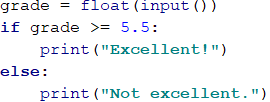#### Testing in Judge System

By pressing the tab key we create a block of code through which a group of commands can be executed. When we have code in if, elif, else (and other structures) and we want to perform a series of operations, we put them in a block after the condition.It is a good practice to use a tab (or four spaces) since this makes the code more readable, neater and cleaner. In this way, we avoid errors during code execution.

Here is an example of bad indentation: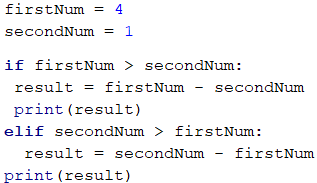The above code will either give an error because it is incorrectly formatted, or its execution will display the wrong result on the console: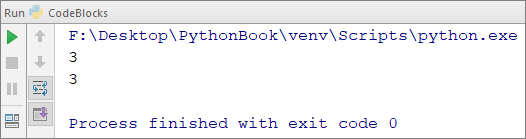With correct indentation: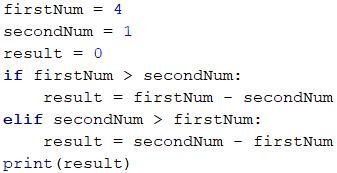The following output will be printed on the console: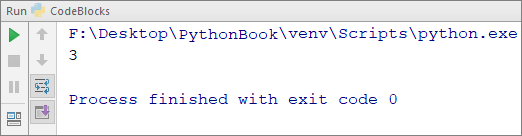### Problem: Even or Odd

Write a program that checks whether a given integer number is even or odd.

The problem can be solved with a single if-else structure and the operator %, which returns the division remainder of two numbers:### Problem: Greater Number

Write a program that reads two integers and outputs the number of higher value between the two.

Our first task is to read the two numbers. After which through the use of a simple if-else structure, in combination with the greater than operator (>), to perform the comparison. We have deliberately blurred parts of the code so that the reader can implement the learned so far.## The Lifetime of a Variable

Every variable has a scope in which it exists, called variable scope. This scope specifies where the variable can be used and accessed. In Python, variables could be used anywhere if they are initialized at least once.

In the example below, on the last line, we try to print the variable my_name, which is defined in the else structure. We will get an error, because in this case the body of the else clause, in which we initialize the variable, is not executed. However, there is no problem printing the variable can_drive because the program entered the body of the if clause and initialized the variable. As you can see the variables can_drive and my_name are colored yellow. This is a warning from PyCharm, that we may get an error. Therefore, we should be careful where we initialize the variables.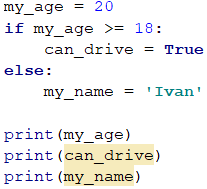## Conditional Chaining

Sometimes we have to do a series of checks, before deciding what actions our program will execute. In such cases we can apply the structure if-elif ... else in series. For this purpose, we employ the following structure:

if condition:
# condition body;
elif condition2:
# condition body;
elif condition3:
# condition body;
…
else:
# else structure body


### Problem: Number 0...9 to Text

Print the digits one through nine in English on the console (the numbers are read from the console). We can take the digit and through a series of conditions print the corresponding English word on the console:

number = int(input())

if number == 1:
print("one")
elif num == 2:
print("two")
elif …:
…
elif num == 9:
print("nine")
else:
print("number too big")


The program logic of the above example sequentially compares the input number with the digits from 1 to 9 with each consecutive comparison being performed only in case the previous result is not true. Eventually, if none of the if conditionals are satisfied, the last else clause is executed.

## Problems: Simple Conditions

To practice the implementation of the conditional constructs if and if-elif we will take a look at a few practical problems.

### Problem: Bonus Score

We are given an integer – several points. Additional bonus points are awarded as per the rules described below. Write a program that calculates the bonus points for the given number and outputs the total points including the bonus.

• If the number is up to 100 inclusive, the bonus points are 5.
• If the number is larger than 100, the bonus points are 20% of the number.
• If the number is larger than 1000, the bonus points are 10% of the number.
• Additional points are awarded as below (added separately from the described above):
• For even numbers -> + 1 p.
• For numbers, ending with 5 -> + 2 p.

Input Output
20 6
26
175 37
212
2703 270.3
2973.3
15875 1589.5
17464.5

#### Hints and Guidelines

We can calculate the base and additional bonus score with a series of if-elif-else statements. For the main bonus points we have 3 cases (the input is less than or equal to 100, it is between 100 and 1000, and finally it is greater than 1000), for the additional bonus score - further 2 cases (whether the number is even or odd):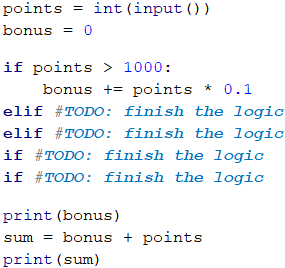Here’s what the solution to the problem might look like:Please note that for this problem the Judge system is set up to ignore any non-number outputs, so we may print explanations along with the number output.

### Problem: Sum Seconds

Three athletes finish with some number of seconds (between 1 and 50). Write a program that reads the times of the contestants and calculates their combined time in "minutes:seconds" format. Seconds are to be printed with a leading zero (2 -> "02", 7 -> "07", 35 -> "35").

Input Output
35
45
44
2:04
22
7
34
1:03
50
50
49
2:29
14
12
10
0:36

#### Hints and Guidelines

Firstly, we sum the three numbers, to obtain the total seconds. As we know that 1 minute = 60 seconds, we should calculate the minutes and seconds in the range 0 to 59:

• If the result is between 0 and 59, we print 0 minutes + calculated seconds.
• If the result is between 60 and 119, we print 1 minute + calculated seconds minus 60.
• If the result is between 120 and 179, we print 2 minutes + calculated seconds minus 120.
• If the seconds are less than 10, we print the number with a leading zero.### Problem: Metric Converter

Write a program, that converts distance between the following 8 units of measure: m, mm, cm, mi, in, km, ft, yd. You may use the conversion table below:

Input measure Output measure
1 meter (m) 1000 millimeters (mm)
1 meter (m) 100 centimeters (cm)
1 meter (m) 0.000621371192 miles (mi)
1 meter (m) 39.3700787 inches (in)
1 meter (m) 0.001 kilometers (km)
1 meter (m) 3.2808399 feet (ft)
1 meter (m) 1.0936133 yards (yd)

The input will consist of three parameters:

• First line: A number.
• Second: Input unit of measure.
• Third: Output unit of measure (for the result).

Input Output
12
km
ft
39370.0788
150
mi
in
9503999.99393599
450
yd
km
0.41147999937455

#### Hints and Guidelines

We take the input data and to the units of measure, we can add the function lower(), which will convert all letters to lower case. As we can see from the conversion table above, we have data for converting only between meters and any other measuring unit. To make the conversion, firstly we must calculate the input measurement in meters. To this effect, we need to create a set of conditionals to determine the input measuring unit and then the output.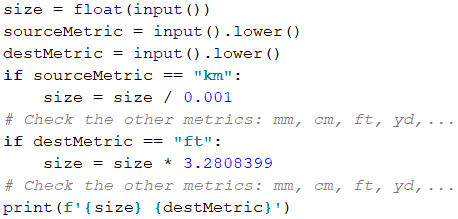## Debugging - Simple Operations With a Debugger

So far, we have written quite a lot of code and oftentimes there have been mistakes, haven't there? Now we can show you a tool to make finding mistakes easier.

### What is "Debugging"?

Debugging is the process of "attaching" to a program's execution, which allows us to follow closely the execution of our program. We can follow line by line the events in our program, what its evaluation route is, what the intermediate values of the declared variables at each step of the execution are, among other useful information and thus allowing us to locate errors - the so-called bugs: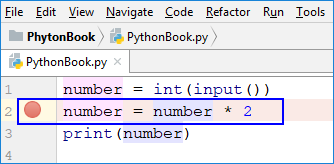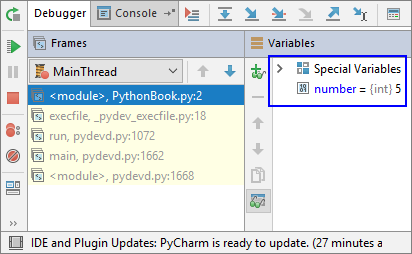### Debugging in PyCharm

By pressing [Shift + F9], we start the program in Debug mode. We move on to the next line of execution with [F7]: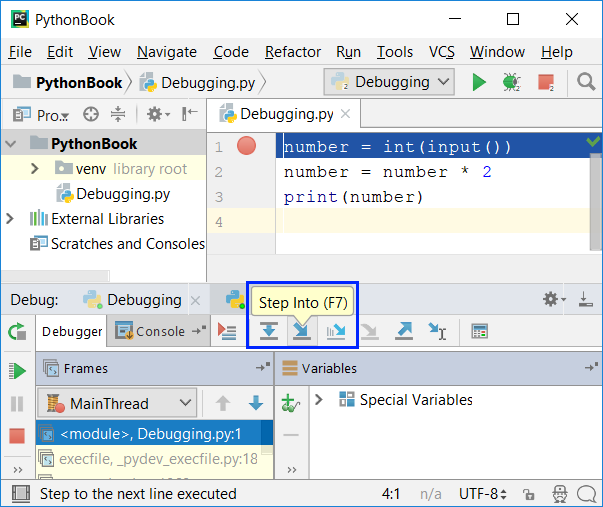With [Ctrl + F8] we create breakpoints, which we can reach directly using [Shift + F9] (when starting the program in Debug mode).

## Problems: Simple Conditions

Now let's practice the lessons learned in this chapter with a few practical exercises.

### Empty PyCharm Solution (Project)

We create empty solution in PyCharm so we can organize the solutions to the tasks from the exercises – each task will be in a separate file and all tasks will be in the same Project.

We start PyCharm and create a new Project: [File] -> [New Project].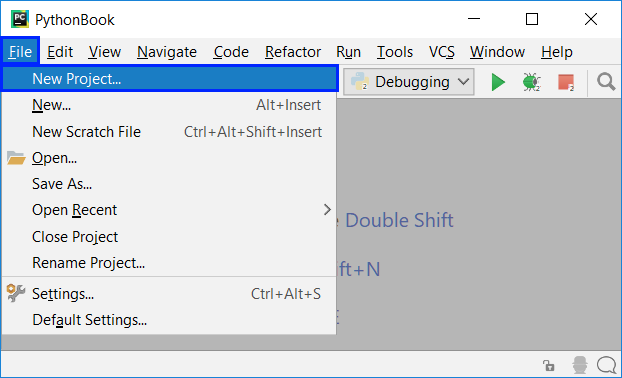Select Pure Python from the field on the left and set the project directory, putting the name of your project in place of the untitled one: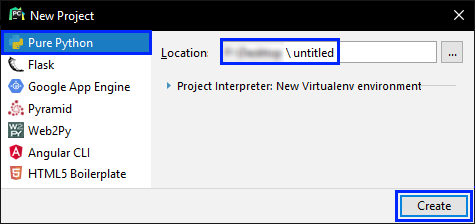Now we have an empty project (no files in it).

Write a program that reads input data from the console - a password (one line of random text) and checks if the input matches the phrase "s3cr3t!P@ssw0rd". If it matches, print "Welcome", otherwise print "Wrong password!".

#### Sample Input and Output

Input Output
s3cr3t!P@ssw0rd Welcome

#### Hints and Guidelines

Use an if-else statement.

### Problem: Number 100...200

Write a program that reads input data from the console - an integer and checks if it is below 100, between 100 and 200 or over 200. Print the appropriate messages as per the examples below.

#### Sample Input and Output

Input Output
95 Less than 100
120 Between 100 and 200
210 Greater than 200

### Problem: Equal Words

Write a program that reads input data from the console - two words and checks if they are the same. A comparison should be case-insensitive and the output should be either "yes" or "no".

Input Output
Hello
Hello
yes
SoftUni
softuni
yes
Soft
Uni
no
beer
vodka
no
HeLlO
hELLo
yes

#### Hints and Guidelines

Before the comparison, both words should be in lower case, so that case (uppercase / lowercase) does not influence the result word = word.lower().

### Problem: Speed Info

Write a program, that reads input data from the console - speed (decimal number) and prints speed information. For speed up to 10 (inclusive), print "slow". For speed over 10 up to 50, print "average". For speed over 50 and up to 150, print "fast". For speed over 150 and up to 1000, print "ultra fast". For any higher speed, print "extremely fast".

#### Sample Input and Output

Input Output
8 slow
49.5 average
126 fast
160 ultra fast
3500 extremely fast

### Problem: Area of Figures

Write a program that reads input data from the console - the measures of a geometric shape and calculates its surface area. There are four types of shapes: square, rectangle, circle and triangle.

The first line of input is the type of shape (square, rectangle, circle, triangle):

• If the shape is a square, the next argument will be one number - the length of its side.
• If the shape is a rectangle, the next argument will be two numbers - the lengths of its sides.
• If the shape is a circle, the next argument will be one number - the radius of the circle.
• If the shape is a triangle, the next argument will be two numbers - its base and the corresponding altitude.

The result should be rounded up to the third decimal point.

Input Output
square
5
25
rectangle
7
2.5
17.5
circle
6
113.097
triangle
4.5
20
45

### Problem: Time + 15 Minutes

Write a program that reads two integers - hours and minutes based on a 24-hour day and calculates what time it will be 15 minutes later. The result should be printed in the following format hh:mm. Hours should always be between 0 and 23, while minutes should always be between 0 and 59. Hours should be written with one or two digits as needed, while the minutes should always be written with two digits - add a leading zero, as needed.

Input Output
1
46
2:01
0
01
0:16
23
59
0:14
11
08
11:23
12
49
13:04

#### Hints and Guidelines

Add 15 minutes and check using a set of conditions. If minutes are over 59 increase the hours by 1 and decrease the minutes by 60. You may handle the case when hours are over 23 similarly. Take care when printing the minutes to add a leading zero where appropriate.

### Problem: 3 Equal Numbers

Write a program that reads 3 numbers and prints whether they are the same ("yes" / "no").

Input Output
5
5
5
yes
5
4
5
no
1
2
3
no

### Problem: Number 0...100 to Text

Write a program that converts numbers in the range of [0 … 100] in text.

Input Output
25 twenty five
42 forty two
6 six

#### Hints and Guidelines

Firstly, we should check for single-digit numbers and if this is the case, print the corresponding word. Then we can check if the number is a double-digit number. These can be printed in two parts: left part (double-digit = number / 10) and right part (single-digit = number % 10). If the number has three digits, then it must be 100 and this can be handled as a special case.